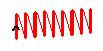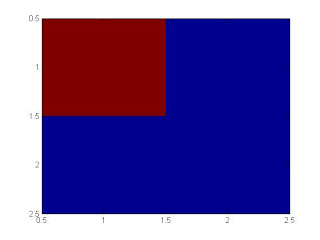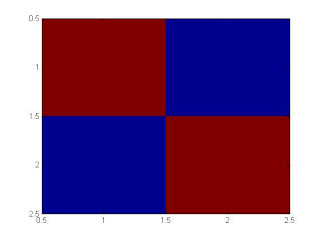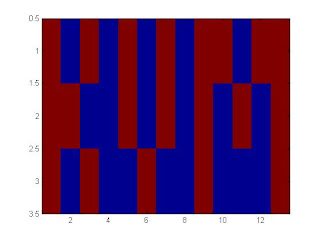## Friday, January 19, 2007

### Quines of the World Unite!

Ladies and Gentlemen, let me introduce our brand new member of the Pantheon, Mr. Jeff Tupper. What has he done to deserve this rich honour ? He invented a Math Quine ! A Quine is a program that reproduces its entire source code. There have been quite a few very interesting ones, but till now I haven't seen anything like a Math Quine. This equation here:whereis the floor function andis the mod function produces this as a graphwhen plotted between
(0 <= x <= 106) and (a <= y <= a + 17) where a = 960939379918958884971672962127852754715004339660 1293066515055 1927170280239526642468964284217435 071812126715378277062335599323728087414430789132 596394133772348785773574982392662971551717371699 516523289053822161240323885586618401323558513604 882869333790249145422928866708109618449609170518 345406782773155170 54053816273809676025656250169 814820834187831638491155902256100036523513703438 744618483787372381982248498634650331594100549747 005931383392264972494617515457283667023697454610 1465599793 3798537483143786841806593422227898388 722980000748404719

[This white space is meant for you to let your lower jaw get back into comfortable equilibrium after it fell (probably) 3 feet to the floor]

AMAZING! After the pure joy slowly attenuated, inspired by this guy whom I mentioned in this post, I set out on reverse engineering this equation, with these goals:

0. Make sure he's not bluffing.
1. Find out how it works.
2. Find out how Mr.Tupper could have thought of it.

First, Let us try to think of a method to verify this inequality. How do we do that? Plot it, of course. Nice, now how do we plot it? I ask because it is an implicit inequality, and so I can't seem to get an expression for y in terms of x. So, we'll go to every point in the domain, and try plugging in the inequality.

How many points? The presence of lots of floor()s in the equation seems to suggest that we can probably get a good enough approximation if we look at only the integer lattice points. How many are there? 105*17, that is trivial for any plotter, so off we plot.

I tried plotting it as it is in Matlab, but it didn't work. Why? The value of n was too large, and matlab was treating it as Inf. So, the for y = n : n + 16 loop wasn't running at all. I tried googling if anybody else had done it, but all I found (Thanks Raghu) was a Maple assignment, no proof it having been tried out anywhere.

So, for the moment, let us believe Mr. Tupper. But, if we haven't got it working ourselves, how are going to find out how it works ?

Let us look at the expression, and see if we can get something from it. The outermost function is a floor(), so the expression can only be an integer. Not much to be gained from that, but why would he put > 0.5 in front of an integer? Ok, lets go ahead. The next function is a mod ( [something] , 2) , that is, the remainder of [something] when divided by 2. Aha !! This value can only be between 0 and 2, 2 not included !!!!! ( mod(3.5,2) == 1.5 by the way ).

But then, we're doing floor() of this number, so the expression can only be ZERO OR ONE! Mr. Tupper is plotting like an LED display !!!! He wants us to go to every point in the domain, check if the expression is 1 or 0 (that is what > 1/2 does; it could as well have been > 0.1 or > 0.9), and plot it if is 1 !!

That still doesn't give us any clue on how he figured out that horrendous number, and how he is controlling every pixel in the map! Looking at the inside of the mod(), we see both x and y appear inside floor()s. What could the reason be? It would mean that for every value of x and y between two integers, the function does not change. But of course! Mr.Tupper is making a _finite_ pixel, of width x=1 and y=1 ! So the size of the LED is fixed now!! Also, we see that y appears only as floor(y/17) and mod(y,17), so there is something fishy about 17. Looking at y being so large, and 17 being so fishy, if we put

y = 17*a + t , a and t are integers,

the expression comes down to:

(I'm writing floor(mod(..,2)) as fm(..))

0.5 < fm( a / 2^(17x + t) )

That 17 still is irritating. What is it doing there ? Hmm, how did we think of plotting this graph again? We said we would go to every point in the domain, and calculate fm. How do we go to every point? You need to represent a 2D matrix as a 1-D array, (1D ordered pairs of all points we will go to). AND BINGO! 17x + t is the very familiar scan pattern! That is exactly how your TV or comp screen draws! For a given x, vary y. Then, go to the next x, vary y, voila !! If Mr. Tupper was thinking on paper, he'd have thought along these lines, literally:But it is more probable he was thinking on a comp, and so he'd have moved _down and up_ instead of up and down, because y increasing downwards is a common convention.

Mr.Tupper's mind is getting clearer and clearer :-)

Now then, that was simply a theory, any proof that was what he did ? If this true, the image must be 17 pixels high. How much is it? Lets check, it is 67 px wide. But that is simply 17*4 - 1 (This 1 pixel row clipping happens lots of times) So Mathworld has simply scaled up the image 4 times ! Clear as a bell!!!

So that is perfectly fine; The mystery still remains, how is this expression lighting up just the right LEDs ? Let us write this expression again:

0.5 < fm ( a / 2^(hx + t) )

So, the whole problem reduces to finding out the value of a, because h is a constant we determine! How is it that the value of a determines everything?

Here, I slightly digressed, and instead of a / 2^(hx + t), I did a * 2^(hx + t) . It essentially does the same job,as you will see. Let us now try to draw this box:Very simple, 2x2 box. What value of a will make the expression draw this?

The first LED should be 1, the other 3 should be 0. For first one to be on, the fm() expression should be 1. For the fm() expression to be 1, the mod([something],2) expression should be 1. Which means [something] should be greater than (we are allowing fractions here) or equal to 2*n + 1, but lesser than 2(n+1). Great. So, plugging in x = 0, y = 0, and requiring fm = 1, we get

a * 2^0 between 2*n + 1 and 2(n+1)

Fine. Next pixel is zero. What does this mean ? fm(..) is zero. This means

mod([something],2) < 1 (strictly lesser)

How? Possible if something < 2n + 1 but something >= 2n. Putting x = 0, y = 1, and fm = 0, we get

a * 2^1 between 2n and 2(n+1)

Note that this n is not the same n as in the previous equation, it is simply some arbitrary n !!

Similarly for the other two points, we get:

a * 2^2 between 2n and 2(n+1)
a * 2^3 between 2n and 2(n+1)

again,
a - 2n+1 - 2n+2
2a - 2m - 2m+1
4a - 2p - 2p+1
8a - 2q - 2q+1

How are we going to find such a? First, let us put n = 0. We get a between 1 and 2. Next, 2a should be between 2m and 2m+1. Does this change the upper or lower bound of a ? If a were to be slightly higher than its present lower bound, ie, 1, then 2a would be slightly higher than 2, which is an even number. What about the upper bound? Aha! If a goes above 1.5, then 2a goes above 3, and the pixel will turn on! We don't want that! So, the upper bound changes to 1.5. So now, we know a is between 1 and 1.5. Let's go ahead. We want 4a between 2p and 2p+1. Does the lower bound change?

Wait a minute! We are seeing a pattern here! We have _set_ the lower and upper bounds keeping in mind that if we multiply them by 2^[the step we are in], we get an integer, either 2p or 2p+1 depending on whether we want that pixel or on off. So, definitely, if we go into the next step, multiplying LB or UB by 2^[the step + 1] will surely give two even numbers! So, if we are going to turn a pixel off, we must keep a between that integer, and the integer + 1. Let me illustrate. We got a as between 1 and 1.5. How? We did 2*1 and 2*1.5 , and found out that if a stayed within the two, it would satisfy all previous conditions. So surely, 4*1 and 4*1.5 will be even integers! 4 and 6, to be precise. If we want that pixel to be off, we just make sure that it does not go above 5, because otherwise fm() would become 1 or higher. So, if we want to turn off a pixel, its upper bound changes !!! So going ahead, for the third pixel to be off, 4a is between 2p and 2p+1, and so the upper bound becomes 1.25. Similarly, for 8a to be between 2q and 2q+1, upper bound becomes 1.125.

So any value between 1.0 and 1.125 should give that pattern.

Does it?
xlimit =2 ;
ylimit =2 ;
map = zeros(xlimit,ylimit);
a = 1.06;
for x = 1 : xlimit
for y = 1 : ylimit
t = floor(mod(a*2^(((x-1)*ylimit + y-1)),2));
if (t > 0.5)
map(x,y) = 1;
end
end
end
imagesc(map);IT DOES! PEACE!!

How about if we want this figure?The first condition is the same, a between 1 and 2. The second pixel is off ( we are counting down and right here), so we get a between 1 and 1.5. Third pixel is off, so a between 1 and 1.25. The fourth pixel is on. If we want it on, we want 8a to be between 2p + 1 and 2p. Just by a similar argument to the one above, we see that the lower bound changes if we want to turn on a pixel ! So, if multiplying a number between 1 and 2 by 8 should give me an odd number + some residue, what should be the min value of that number? Obviously, 1.125 ! So, 1.125 to 1.5 is the range for a to be in, and anything in this range will give the desired output. Does it?Yes, it does !!

By now, the algorithm to get the value for a is clear; Off pixels will change the upper bound and on pixels will change the lower bounds. So I wrote a small Matlab code, and tried making it output by name. The input matrix I gave was:

target = [
1 0 1 0 1 0 1 0 1 1 0 1 1;
1 1 0 0 1 0 1 0 1 0 1 0 1;
1 0 1 0 0 1 0 0 1 0 0 0 1;
];

From this, it calculated a to be between 1.3349100840896426234394311904907226562500 and 1.334910084093280602246522903442382812500

I chose (lower+higher)/2 and plotted it, and voila!!So the equation of my name is:

0.5 < fm(1.33491008409146160 * 2^(3x + y))
x from 0 to 17, y from 0 to 3

I tried doing a few others, but lower and higher start differing by _very_ (50th decimal place) small amounts. I tried Raghu's name, but got only 'Ra'. So, choosing a * 2^.. will increase the number of decimal digits in a, and similarly, a / 2 ^.. will increase the digits. So, that 541 digit number is all there is to it!

My God, if this was so much fun reverse engineering, I can't imagine how awesome it'd have been to come up with it in the first place. The choice of 2^.. as the function that would be evaluated at every point is PURE genius!

Here is the Matlab code. Put your LED pattern in the 'target' matrix, and look for the value of 'a'. My Matlab distro can't go beyond some 20 elements in the target, how does yours fare?

target = [
1 0 1 0 1 0 1 0 1 1 0 1 1;
1 1 0 0 1 0 1 0 1 0 1 0 1;
1 0 1 0 0 1 0 0 1 0 0 0 1;
];
s = size(target);
xlimit = s(1);
ylimit = s(2);
map = zeros(xlimit,ylimit);

lower = 0.00;
higher = 2.00;
count = 0;
for i = 1 : xlimit
for j = 1 : ylimit
mul = 2^count;
if(target(i,j) == 1)
lower = lower + 1/mul;
else
higher = higher - 1/mul;
end
count = count + 1;
end
end

a = (lower+higher)/2;a

for x = 1 : xlimit
for y = 1 : ylimit
t = floor(mod(a*2^(((x-1)*ylimit + y-1)),2));
if (t > 0.5)
map(x,y) = 1;
end
end
end
imagesc(map);

:-)

Update: Here is Pota, with a = 1.8666940472197382All equations in this post are from this Mathworld article.

pota said...

KVM....
you just the gyad!Now to get this onto digg
hmmm

KB said...

gawd!

Makam said...

themiddler said...

Wow! Can't say I have completely understood it.
But great way of presenting the concept... quite captivating!

themiddler said...

AWESOME! Now I can falunt my 'a'!

And if I meet someone new, "Hey, what's your 'a'?" Could be an interesting pickup line! :D

CB said...

Macha,
I didn't even read the post da!
It sounds insane! ;-)Anonymous said...

Gawd wonly!

- Pops

Google's problems may start seeming trivial to you at once!
Took 2 hours to understand a reasonable amount of it. But yet again, our Quake 3 engine post makes a reappearance in a different figure altogether.
And 'Just the Jawd' also makes an appearance here, only I will have to put it in caps, with fluorescent font colour, bold, italics, underlined, flashing with lights all around.
And fitting tribute to the Pota too :)
Speaking of Quines, I'm rather too sure you must have checked this. The page has a really bell-ringing section on the actual recovery of data that has to be the program content itself, to be printed. This is called bootstrapping(remember Vitto?). The connection with the bootstrapping we learned, though, is still vague.Anonymous said...

Is it really a quine though? Seems to be it would have to output that huge seed value somehow as well to qualify. Not saying that it isn't amazing though!

Mohan K.V said...

@all : Thank you good sirs!

@Anonymous:

A most profound question. Maybe Mr.Tupper would argue this way: It is a Quine if you know where to look. After all, you _need_ to know that a javascript quine puts its output in a browser; a c++ text quine puts it to stdout and not, say, to an X window, so, knowing where to look doesn't seem part of the definition of a quine. So, all the x and y limits tell you is where to look :-)

Worst abuse of rules, anyone ?

amrut said...

You are a god!

wolverine said...

kv... calling u gawd is an awful cliche.. still ur THE MAN!!!!

essen arren said...

Brilliant!

The next challenging thing would be to write a code which can _plot_ itself!?

Arun 'Randi' said...

i must appreciate you for a couple of things:
1.your inquisitive nature to solve such a problem(weird to most homo-sapiens)
2.your patience to write such a super looong article

However, i lost track half-way through the post! :( :D

Shankar said...

wow!!!! that was something!

bumps said...

Awesome stuff!!!!

Tejas said...
This comment has been removed by a blog administrator.
dushy said...

Nice!
Thats too less a say for you have written such a long Funda post!
Get going! :)Anonymous said...

A true Quine equation from Mr. Tupper has been circulating for a few days. Perhaps you could explain that one too?

amrit said...

Awesome!

parseval said...

Your explanation is absolutely freaking awesome! Mind blowing!

It's the most original and enjoyable thing I've read in a long time.

Have you thought of publishing a paper on this? ;)

Arunn said...

Mohan

see this post at my blog.

pick it up at your blog, if you feel like it.